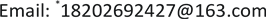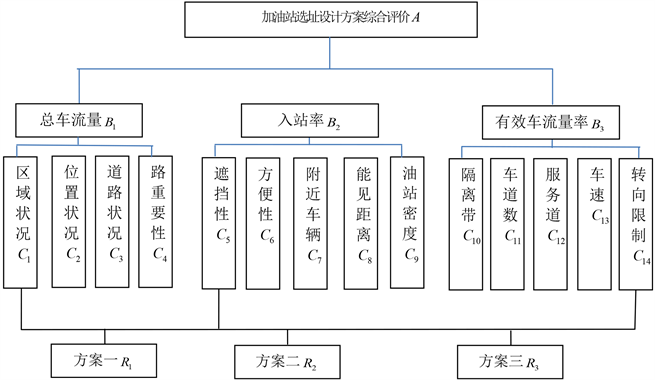﻿ 模糊决策在加油站选址中的应用 The Application of Fuzzy Decision in the Gas Station Location

Vol.07 No.06(2018), Article ID:25481,6 pages
10.12677/AAM.2018.76082

The Application of Fuzzy Decision in the Gas Station Location

Fanghui Li*, Yebing Zhang

College of Science, North China University of Technology, BeijingReceived: May 24th, 2018; accepted: Jun. 12th, 2018; published: Jun. 19th, 2018ABSTRACT

In this paper, choosing fourteen main influencing factors with respect to total traffic flow, the efficiency and the inbound rate, the index system of comprehensive evaluation is established. Using the analytic hierarchy process, the weight matrix of different influence factors is obtained. The optimal proposal is determined according to the fuzzy decision theory.

Keywords:Fuzzy Decision, Analytic Hierarchy Process, Gas Station Location, Scheme Evaluation1. 引言

2. 层次分析法确定权重

2.1. 建立层次结构图Figure 1. Gas station selection scheme evaluation hierarchy

2.2. 构造判断矩阵

2.3. 一致性检验

0.58，0.90，1.12，1.24，1.32，1.41，1.45。当 $RI<0.01$ 时，判断矩阵具有满意一致性。

3. 模糊综合评判进行优选

$A=\left(0.3576,0.0381,0.0484,0.1427,0.0105,0.0835,0.0947,00.0161,0.0346,0.0062,0.0662,0.0364,0.0182,0.0102\right)$ 建立因素集合U的评语集合V = {很好，较好，一般，较差}，并相应的记分为{10,8,6,4}。对各因素进行

${B}_{1}=A{B}_{1}=\left(0.3511,0.2257,0.3399,0.0831\right)$ , ${V}_{1}={b}_{11}{v}_{1}+{b}_{12}{v}_{2}+{b}_{13}{v}_{3}+{b}_{14}{v}_{4}=7.6884$

${B}_{2}=A{B}_{2}=\left(0.5433,0.2873,0.1490,0.0203\right)$ , ${V}_{2}={b}_{21}{v}_{1}+{b}_{22}{v}_{2}+{b}_{23}{v}_{3}+{b}_{24}{v}_{4}=8.7066$

${B}_{3}=A{B}_{3}=\left(0.4781,0.2554,0.1933,0.0729\right)$ , ${V}_{3}={b}_{31}{v}_{1}+{b}_{32}{v}_{2}+{b}_{33}{v}_{3}+{b}_{34}{v}_{4}=8.2756$

4. 结论

2018年北京市大学生科学研究与创业行动计划，北京市教育委员会(6943.KM201710009012)。

The Application of Fuzzy Decision in the Gas Station Location[J]. 应用数学进展, 2018, 07(06): 685-690. https://doi.org/10.12677/AAM.2018.76082

1. 1. 杨德锋. 加油站选址研究[J]. 中国石油大学学报(社会科学版), 2006, 22(3): 20-23.

2. 2. 刘砚田. 工程经济[M]. 西安: 西安交通大学出版社, 1988.

3. 3. 陈水利, 李敬功, 王向公. 模糊集理论及其应用[M]. 北京: 科学出版社, 2009.

NOTES

*通讯作者。